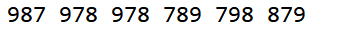•  我们首先定义了getmax函数，将一个任意三位数重新排列，返回三位的最大值  然后分别定义了max,min,middle函数  最终实现返回三位的最大值的功能  //函数的功能：将一个三位进行重新排列得到最大的...
设计一个程序，将一个三位数重新组合得到最大的三位数，比如472的各位数字之和就是742。
我们首先定义了getmax函数，将一个任意三位数重新排列，返回三位数的最大值
然后分别定义了max,min,middle函数
最终实现返回三位数的最大值的功能

//函数的功能：将一个三位数进行重新排列得到最大的数
//函数的名字：getmax
//函数的参数：一个待判断的数
//函数的返回值：三位数的最大值
var num =
var num1
= Math.floor(num /
100);
var num10
= Math.floor(num /
10) % 10;
var num100
= num % 10;
var max
= Math.max(num1,
num10, num100);
var min
= Math.min(num1,
num10, num100);
var middle
= Middle(num1,num10,
num100);
var res
= max *100+middle
*10 + min;
return res;
}
/*功能：得到三个数中最大的数
名字：max
参数：三个数
返回值：最大的那个数
*/function max(num1,num2,num3){
var max
= num1;
if(max
< num2){
max =num2;
}
if(max
<num3){
max =num3;
}
return max;
}
/*功能：得到三个数中最小的数
名字：min
参数：三个数
返回值：最小的那个数
*/
function min(num1,num2,num3){
var min
= num1;
if(min
> num2){
min= num2;
}
if(min
>num3){
min= num3;
}
return min;
}
/*功能：得到三个数的中间数
名字：middle
参数：三个数
返回值：中间的那个数
*/
function Middle(num1,num2,num3) {
if (num1== Math.max(num1,num2, num3)) {
returnMath.max(num2,num3);
} elseif (num2 ==
Math.max(num1, num2, num3)) {
returnMath.max(num3,num1);
} elseif (num3 ==
Math.max(num1, num2, num3)) {
returnMath.max(num1,num2);
}
}


展开全文• var n = parseInt(window.prompt(“input”));------------网页可输入弹出框 var a, b, c; a = n % 10; ----------------------------------提取... ----------------------提取 三位数中的十位 b = (n - (n...
2019.6.21  更新：
var n = parseInt(window.prompt('input'));
var one,
two,
three;
one = n % 10;
two = n % 100 - one
three =n-(n % 100)
n = one*100 + two+ three/ 100;
document.write( n );

var n = parseInt(window.prompt(“input”));------------网页可输入弹出框
var a,
b,
c;
a = n % 10; ----------------------------------提取三位数中的个位数
c = (n % 100)-a ; ----------------------提取 三位数中的十位数
b = n - (n %100);--------------------提取三位数中的百位数
var m = a*100 + c + b/100;
document.write(m);
例如输入456；
1.提取个位数：
456 % 10 = 6  = a
转化为百位数：
6 * 100 = 600
2.提取十位数：
(456  % 100) - 6 = 50 =c
转化为十位数：
50
3.提取百位数
456 - (456 %100) = (456 - 56)= 400 = b
转化为个位数：
400 /100 = 4
输出：m
m = a100 + c + b/100;
m = 6100 + 50 + 400/100
=600+50+4
=654
简洁版：
var n = parseInt(window.prompt(‘input’)),
//提取个位变百位
b = (n % 10) * 100;
//提取十位数
a = (n % 100) - (n % 10);
//提取百位数 变成个位数
c = (n - (n % 100) ) / 100;
document.write(b + a + c);


展开全文js 反向输出数字
• (1)打印由7，8，9三个组成的三位数，要求该三位数任意两位数字不能相同； (2)打印格式最后的三位数字以空格分隔，如789 798 879 897 978 987。 注：要求使用StringBuilder来完成 */ public class Demo3 { ...

/*
分析以下需求，并用代码实现：
(1)打印由7，8，9三个数组成的三位数，要求该三位数中任意两位数字不能相同；
(2)打印格式最后的三位数字以空格分隔，如789 798 879 897 978 987。
注：要求使用StringBuilder来完成
*/
public class Demo3 {
public static void main(String[] args) {
int number;
int count = 0;
while (count != 6) {
StringBuilder sb = new StringBuilder();
Random r = new Random();
number = r.nextInt(3) + 7;
sb.append(number);
number = r.nextInt(3) + 7;
sb.append(number);
number = r.nextInt(3) + 7;
sb.append(number);
if (sb.charAt(0) != sb.charAt(1) && sb.charAt(0) != sb.charAt(2) && sb.charAt(1) != sb.charAt(2)
) {
System.out.print(sb + " ");
count++;
}

}
}
}展开全文• 任意三位数打乱次序后的最大值 Time Limit: 1 Sec Memory Limit: 128 MB 64bit IO Format: %lld Description 任意输入一个三位整数，再把它的次序打乱重新组合一个新的三位整数，使其值最大。   Input ...
求任意三位数打乱次序后的最大值

Time Limit: 1 Sec  Memory Limit: 128 MB   64bit IO Format: %lld

Description

任意输入一个三位整数，再把它的次序打乱重新组合一个新的三位整数，使其值最大。

Input

包含多组测试数据，每组测试数据占一行，每行1个3位数。

Output

每组测试数据输出占一行，输出1个整数。

Sample Input123

Sample Output

321

题目分析：

为什么不用 %c 来完成这项任务呢？

无脑排列组合，启动！

#include<stdio.h>

int main()
{
char a,f,b,c;
while(scanf("%c%c%c",&a,&b,&c)!=EOF)
{
getchar();
if(a>=b && b>=c)
printf("%c%c%c\n",a,b,c);
else if(a>=c && c>=b)
printf("%c%c%c\n",a,c,b);
else if(b>=c && c>=a)
printf("%c%c%c\n",b,c,a);
else if(b>=a && a>=c)
printf("%c%c%c\n",b,a,c);
else if(c>=a && a>=b)
printf("%c%c%c\n",c,a,b);
else if(c>=b && b>=a)
printf("%c%c%c\n",c,b,a);
}
return 0;
}



展开全文• Python程序：任意输入一个三位数，然后把三位数的位置反转输出。 第一种方法，把输入的三位数百位、十位和个位依次表示出来，然后将个位数字和百位数字进行交换。 #输入number = 123,输出321 number = int(input('请...python 列表 字符串
• 从控制台输入任意一个三位，倒叙输出这个三位 import java.util.Scanner; public class test{  public static void main (String [] args){  Scanner sc=new Scanner (System.in);...请输入任意位三位数：...Java
• public class TestShu1 {private static int[] a={4, 2, 3, 9};...//一个组成三位数 private static int hunNum=0;//组成的三位数 //bai-百位, shi-十位, ge-个位public static int getHuNum(intJava
• 题目：求整数的任意次方最后三位数！347的72次方最后三位数是241.#include&lt;stdio.h&gt; int main() { int x, y, p, n; printf("说明：底数为x,幂为y，请输入:\n"); printf("x=");...c语言 精度问题
• 任意输入一个三位整数，再把它的次序打乱重新组合一个新的三位整数，使其值最大。 输入 输入只有一行，包括1个整数。 输出 输出只有一行（这意味着末尾有一个回车符号），包括1个整数。 样例输入 470 样例...Pascal
• 题目 - 对于一个任意三位自然数X，编程计算其各个位上的数字之和S。 刚拿到题目，能想的出几种做法: 方法1: 转换为文本，在 foreach 中通过 Java 的 String.codePointAt(int indexjava 算法
• 对于一个任意三位自然数X，编程计算其各个位上的数字之和S。 输入 输入一行，只有一个整数x(100 输出 输出只有一行，包括1个整数 样例输入 123 样例输出 6   C++代码 #inc lude using namespace ...c++
• //精度问题！ /* =============================================...求整数的任意次方最后三位数！347的72次方最后三位数是241. ================================================================== */ #includeC语言 精度问题
• 求一个整数任意次方的最后三位数，即x^y的最后三位数，要求x，y从键盘输入 解析： 取最后三位数，我们首先想到的肯定是用取余运算，如果直接将运算结果来进行取余的话就会有越界的危险，因此，我们可以...C语言 算法
• #include #include void main() { int a,i,b; long sum,n,s; scanf("%d%d",&a,&b); sum = pow(a,b); for ( i=3; i>0; i-- ) { s = sum/pow(10,i-1); n = s%10;...}include
• 回归正题，当时学循环时以为水仙花就是一个各位数字立方和等于它本身的三位数，但实际任意位数都可求水仙花，此处贴下我的代码。 #include&amp;amp;amp;amp;amp;amp;lt;stdio.h&amp;amp;amp;amp;amp;amp...c语言
• ## 三位数的数位分离

千次阅读 2019-04-14 17:48:06
1004: 三位数位分离 题目描述 从键盘输入一个任意三位正整数，分别求出其个位、十位和百位上的数字。 输入 输入任意的一个三位正整数。 输出 依次输出个位、十位、百位上的数字。以空格间隔，但最后一个数据的...
• 法一： var n = parseInt(prompt('输入一个三位数')); var a, b, c; a = (n - (n % 100)) / 100; //取百位 c = n % 10;JavaScript 字符串反转
• Python实例002：n个数字能组成多少个互不相同且无重复数字的三位数，分别是多少 n个数字能组成多少个互不相同且无重复数字的三位数，分别是多少 #!/usr/bin/env python3 # -*- coding:utf-8 -*- List = input('请...Python实例
• 问题描述:求一个整数的任意次方的最后三位数,即x^y的最后3位数 分析： 3.代码实现： #include&lt;stdio.h&gt; #include&lt;math.h&gt; void sum(int x,int y) { int sum; sum=pow(x,y); if...
• 若是水仙花，则输出"该三位数是水仙花！"，否则输出“该三位数不是水仙花！”。 提示：所谓"水仙花"是指一3位数，其各位数字立方和等于该本身。 例如：153是一个水仙花，因为153=1+125+27。 输入格式: %...
• 编写函数计算任意位数的黑洞（初学） 黑洞是指这样的整数： 由这个数字每数字组成的最大减去每数字组成的最小数仍然得到这个自身。例如3黑洞是495，因为954-459=495，4数字是6174，因为7641-...Python
• #include int main() { int i,x,y,z=1; scanf("%d%d",&x,&y);//输入底数和幂 for(i=1;i;i++) ...如何避免产生越界问题是本题的关键，这里求次方时每一次都取其后三位，这样就不会产生越界问题。
• 任何一个数字不全相同的三位数，经过有限次“重排求差”操作，总会得到495。 “重排求差”操作是将组成一个的各位数字重排得到的最大减去 最小数。例如107，“重排求差”操作序列为：710-17=693，963-369=594...数学
• var a = prompt("请输入一个三位数"); var b = a%10 //个位数字 var c = (a%100)/10 var d = Math.floor(c)//十位数字 var e = (a/100) var f = Math.floor(e)//百位数字 var g = b+d+f al
• 1004：三位数位分离 题目描述 从键盘输入一个任意三位正整数，分别求出其个位、十位和百位上的数字。 输入 输入任意的一个三位正整数。 输出 依次输出个位、十位、百位上的数字。以空格间隔，但最后一个...java C ZZULIOJ
• C++：三位数的逆序 问题描述 输入一个三位数，输出它的逆序，比如输入123输出321。 输入描述 一个三位数 输出描述 一个三位数（输入三位数的逆序） 输入示例 123 输出示例 321 #include &amp;lt;iostream&...C++ 入门 C++题库
• 编程求一个整数任意次方后的最后三位数，即求x^y的最后三位数，x和y的值由键盘输入。c语言 python...